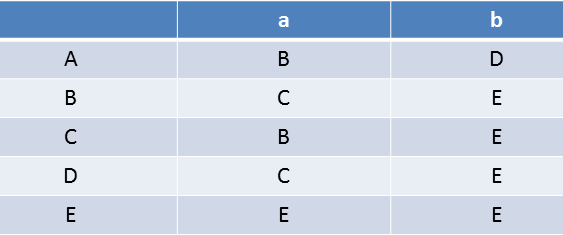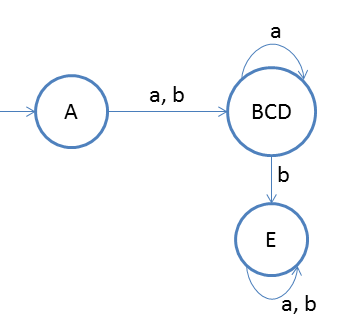# Minimization of DFA Example 2

Minimize the below DFA using partition method for given transition table.### 0 equivalent

[A,B,C,D] and [E] (final and non-final)

### 1 equivalent

Check for A with B, C and D, you will find that B, C, and D should be separated from A
So sets will be: [A], [B], [C], [D] and [E]
But chech whether B, C and D could be combined together
Note:Check in 0 equivalent to divide into sets
Check B and C first: as the o/p of these two states is in same set so they will stay together.
Check for B and D: as the o/p of these two states is in same set so they will stay together.
Check for C and D: as the o/p of these two states is in same set so they will stay together.
So the final 1 equivalent will be
[A], [B,C,D] and [E]

### 2 equivalent

try to split B, C, and D but the output of any of the two states lie in same state. So we stop here and will create new DFA